# Lottery

The lottery is 60000 elk in which 6200 wins. What is the probability that the purchase of 12 elks won nothing?

Result

p =  27 %

#### Solution:

$p = 100 \cdot \ (1 - 6200/60000)^{ 12 }; \doteq 27.013 = 27 \%$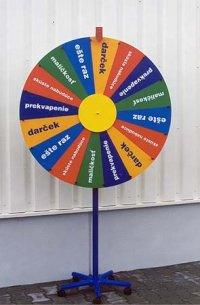Our examples were largely sent or created by pupils and students themselves. Therefore, we would be pleased if you could send us any errors you found, spelling mistakes, or rephasing the example. Thank you!

Leave us a comment of this math problem and its solution (i.e. if it is still somewhat unclear...):Be the first to comment!Tips to related online calculators
Check out our ratio calculator.
Would you like to compute count of combinations?

## Next similar math problems:

1. CardsSuppose that are three cards in the hats. One is red on both sides, one of which is black on both sides, and a third one side red and the second black. We are pulled out of a hat randomly one card and we see that one side of it is red. What is the probab
2. BallsFrom the urn in which are 7 white balls and 17 red, gradually drag 3-times without replacement. What is the probability that pulls balls are in order: red red red?
3. Balls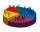We have n identical balls (numbered 1-n) is selected without replacement. Determine 1) The probability that at least one tensile strength number coincides with the number of balls? 2) Determine the mean and variance of the number of balls, which coincides.
4. Medicine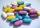We test medicine on 6 patients. For all drug doesn't work. If the drug success rate of 20%, what is the probability that medicine does not work?
5. FamilyWhat is the probability that a family with 7 childrens have: exactly 5 girls? 7 girls and 0 boys? Consider the birth probability of a girl is 48.69% and boy 51.31%.
6. Today in schoolThere are 9 girls and 11 boys in the class today. What is the probability that Suzan will go to the board today?
7. Class - boys and girls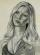In the class are 60% boys and 40% girls. Long hair has 10% boys and 80% girls. a) What is the probability that a randomly chosen person has long hair? b) The selected person has long hair. What is the probability that it is a girl?
8. CardsThe player gets 8 cards of 32. What is the probability that it gets a) all 4 aces b) at least 1 ace
9. Three shooters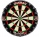Three shooters shoot, each one time, on the same target. The first hit the target with a probability of 0.7; second with a probability of 0.8 and a third with a probability of 0.9. What is the probability to hit the target: a) just once b) at least once
10. Normal distribution GPA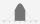The average GPA is 2.78 with a standard deviation of 4.5. What are students in the bottom the 20% having what GPA?
11. Theorem proveWe want to prove the sentence: If the natural number n is divisible by six, then n is divisible by three. From what assumption we started?
12. CandiesIn the box are 12 candies that look the same. Three of them are filled with nougat, five by nuts, four by cream. At least how many candies must Ivan choose to satisfy itself that the selection of two with the same filling? ?
13. ShootersIn army regiment are six shooters. The first shooter target hit with a probability of 49%, next with 75%, 41%, 20%, 34%, 63%. Calculate the probability of target hit when shooting all at once.
14. SequenceFind the common ratio of the sequence -3, -1.5, -0.75, -0.375, -0.1875. Ratio write as decimal number rounded to tenth.
15. Tenth memberCalculate the tenth member of geometric sequence when given: a1=1/2 and q=2
16. GP - 8 itemsDetermine the first eight members of a geometric progression if a9=512, q=2
17. Geometric sequence 4It is given geometric sequence a3 = 7 and a12 = 3. Calculate s23 (= sum of the first 23 members of the sequence).